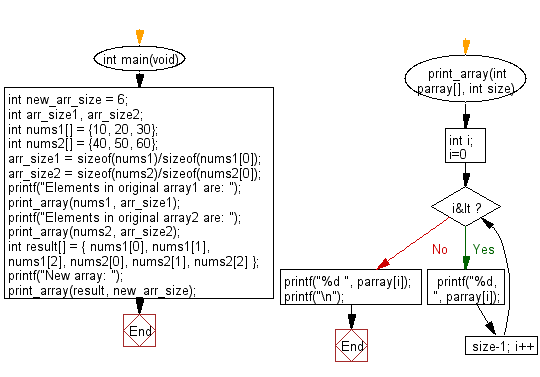﻿ C Program: Array from two array of integers of length 3# C Exercises: Create a new array from two given array of integers of length 3

## C-programming basic algorithm: Exercise-47 with Solution

Write a C program to create a new array from two given array of integers of length 3.

C Code:

``````#include <stdio.h>
#include <stdlib.h>
int main(void){
int new_arr_size = 6;
int arr_size1, arr_size2;
int nums1[] = {10, 20, 30};
int nums2[] = {40, 50, 60};
arr_size1 = sizeof(nums1)/sizeof(nums1);
arr_size2 = sizeof(nums2)/sizeof(nums2);
printf("Elements in original array1 are: ");
print_array(nums1, arr_size1);
printf("Elements in original array2 are: ");
print_array(nums2, arr_size2);
int result[] = { nums1, nums1, nums1, nums2, nums2, nums2 };
printf("New array: ");
print_array(result, new_arr_size);
}
print_array(int parray[], int size)
{
int i;
for( i=0; i<size-1; i++)
{
printf("%d, ", parray[i]);
}
printf("%d ", parray[i]);
printf("\n");
}
``````

Sample Output:

```Elements in original array1 are: 10, 20, 30
Elements in original array2 are: 40, 50, 60
New array: 10, 20, 30, 40, 50, 60
```

Pictorial Presentation:Flowchart:C Programming Code Editor:

What is the difficulty level of this exercise?

Test your Programming skills with w3resource's quiz.

﻿

## C Programming: Tips of the Day

Why doesn't a+++++b work?

printf("%d",a+++++b); is interpreted as (a++)++ + b according to the Maximal Munch Rule!.

++ (postfix) doesn't evaluate to an lvalue but it requires its operand to be an lvalue.

! 6.4/4 says the next preprocessing token is the longest sequence of characters that could constitute a preprocessing token"

Ref : https://bit.ly/3fdldUT